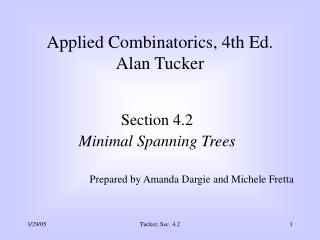# Section 4.2 Minimal Spanning Trees Prepared by Amanda Dargie and Michele Fretta - PowerPoint PPT PresentationDownload PresentationSection 4.2 Minimal Spanning Trees Prepared by Amanda Dargie and Michele Fretta

Section 4.2 Minimal Spanning Trees Prepared by Amanda Dargie and Michele Fretta
Download Presentation## Section 4.2 Minimal Spanning Trees Prepared by Amanda Dargie and Michele Fretta

- - - - - - - - - - - - - - - - - - - - - - - - - - - E N D - - - - - - - - - - - - - - - - - - - - - - - - - - -
##### Presentation Transcript

1. Applied Combinatorics, 4th Ed.Alan Tucker Section 4.2 Minimal Spanning Trees Prepared by Amanda Dargie and Michele Fretta Tucker, Sec. 4.2

2. Recall • Recall:A spanning tree of a graph G is a subgraph of G that is a tree containing all vertices of G. 3 2 3 7 2 4 2 6 3 6 9 1 5 2 1 11 Tucker, Sec. 4.2

3. Definition • A minimal spanning tree in a network is a spanning tree whose sum of edge lengths k(e) is as small as possible. 3 2 3 7 2 4 2 6 3 6 9 1 5 2 1 12 Tucker, Sec. 4.2

4. Kruskal’s Algorithm • Let T be a minimal spanning set (which is initially empty). • Add to T the shortest edge that does not form a circuit with edges already in T. • Repeat adding edges in this manner until set T has n-1 edges Tucker, Sec. 4.2

5. k 1 7 3 2 a u 4 3 2 5 7 q 5 1 2 l 3 g v b 6 7 3 2 8 3 h 4 m 10 r 1 c w 2 3 5 4 3 7 i 8 n 8 s 2 d x 4 2 5 4 3 9 5 6 2 e y Example f p • First include all 3 edges of length 1: (a, f), (l, q), (r, w) • Next add all the edges of length 2: (a, b), (e, j), (g, l), (h, i), (l, m),(p, u), (s, x), (x, y) • Next add almost all the edges of length 3: (c, h), (d, e), (k, l), (k, p),(q, v), (r, s), (v, w),but not (w, x) unless (r, s) were omitted [if both were present we would get a circuit containing these 2 edges together with edges (r, w)and (s, x) • Next add all the edges of length 4 (f, g), (h, m), (c, d), (n, o), (s, t) • Finally add either (m, n)or (o, t)to obtain a minimal spanning set X t o j Tucker, Sec. 4.2

6. Prim’s Algorithm • Let T be a minimal spanning tree, initially including any one edge of shortest length. • Add to T the shortest edge between a vertex in T and a vertex not in T. • Repeat adding edges in this manner until T has n-1 edges. Tucker, Sec. 4.2

7. k 1 7 3 2 a u 4 3 2 5 7 q 5 1 2 l 3 g v b 6 7 3 2 8 3 h 4 m 10 r 1 c w 2 3 5 4 3 7 i 8 n 8 s 2 d x 4 2 5 4 3 9 5 6 2 e y Example f p • There are 3 edges of length 1: (a, f), (l, q), (r, w) • Suppose we pick (a, f) • Next add (a, b), of length 2 • Then (f, g), of length 4 • Then (g, l), (l, q), (l, m) and so forth • The next-to-last addition can be either (m, n), (o, t) (both of length 5) – in this case we’ll use (m, n) • Then follow with (n, o) o t j Tucker, Sec. 4.2

8. Theorem • Prim’s algorithm yields a minimal spanning tree. Proof • Assume that all edges have different lengths. • Let T'be a minimal spanning tree chosen to have as many edges as possible in common with T* (which will be constructed by Prim’s algorithm). Tucker, Sec. 4.2

9. Proof (cont’d) Tucker, Sec. 4.2

10. b a Proof (cont’d) Tucker, Sec. 4.2

11. b a Proof (cont’d) Tucker, Sec. 4.2

12. b a Proof (cont’d) Tucker, Sec. 4.2

13. a d 3 c 7 b 4 8 3 2 g e 5 5 f For the class to try:The red numbers indicate the value of each edge.Find a minimal spanning tree. 5 Hint: use Kruskal’s Algorithm Tucker, Sec. 4.2

14. Solution First, add (c,f) to T Then, add (a,b) and (d,g) Then, add (a,e) Then add (e,f) and (f,g). Don’t add (b,e), because we can’t have a circuit! a d 3 c 7 b 4 8 3 5 2 g e 5 5 f Tucker, Sec. 4.2